We want to look at filters acting on surfaces. The prinicple is the same as those acting on 1D or 3D data. But I especially want to revisit and extend an article I wrote several years ago on smoothing surfaces — Hall, M (2007). Smooth operator: smoothing seismic horizons and attributes. The Leading Edge 26 (1), January 2007, p 16–20. doi:10.1190/1.2431821. I didn't realize it at the time, but that article had some deficiencies:

• It used proprietary data
• It used proprietary software (Landmark's PowerCalculator tool)
• Though I offered to share my scripts, they were not readily available

In this IPython Notebook, I will fix some of these problems. Open data, open software, all on the web, and all CC-BY-SA. I hope you find it interesting and/or useful.

Here's the plan of attack:

• Build a kernel (the filter pattern)
• Run the kernel over the data; there are a couple of ways of doing this
• Look at the output and compare to the input

# Exporting some data¶

First, we'll find our data in OpendTect. We will use the open-access Penobscot dataset from offshore Nova Scotia, quite near my house. This is a great dataset for lots of reasons, but especially because it is freely available and can be shared in almost any way you like. You can download it from the Open Seismic Repository. The data is owned by the Nova Scotia Deptartment of Energy and licensed CC-BY. I used OpendTect software to load the data, then export one of the horizons that comes with the data, a shallow horizon called Horizon B. I exported the whole thing as an inline,crossline (as opposed to x,y) ASCII file.Using NumPy, we can easily read the data into a Python variable called data. NumPy is just a Python library (a bunch of commands, basically) that makes mathematics on big datasets faster. We could use Python's standard tools, but why make things more difficult for ourselves? To use a library like NumPy, we have to import it first.

In :
import numpy
print data[:10]

[[ 1003.          1010.           926.39195919]
[ 1003.          1011.           925.13644695]
[ 1003.          1012.           924.19928312]
[ 1003.          1013.           923.78389835]
[ 1003.          1014.           923.74902964]
[ 1003.          1015.           923.5907793 ]
[ 1003.          1016.           923.72643948]
[ 1003.          1017.           923.48623276]
[ 1003.          1018.           923.40993881]
[ 1003.          1019.           922.38986492]]


The square bracket notation is called a 'slice'; it gives us the first 10 rows of data. Slicing, and the closely related indexing action, are very useful — and you'll see them a lot in Python code.

As expected, we have inline, xline, and two-way time.

Notice that we only exported values where there is data — inlines 1003 to 1597, and xlines 1009 to 1471. We know from OpendTect that the extents of the survey data are: inlines 1000 to 1600, and xlines 1000 to 1481... so null samples were omitted and we need the inline and xline values (aside: otherwise we could just take the third column and reshape it). Also notice that the first row in our dataset is near the lower-left corner of the survey.

To keep things easy later on, we'll use the extents of the horizon from the OpendTect export dialog (above), and preserve this shape.

In :
inlines = 1597 - 1003 + 1
xlines = 1471 - 1009 + 1

print inlines, 'inlines'
print xlines, 'crosslines'

595 inlines
463 crosslines


So we'll want a data object — an array — that has 595 rows and 463 columns. We'll make that in a minute.

Mapping our data into that array will be much easier if we convert those inlines and crosslines to 'coordinates', called indices, in the array. We can use slicing to do math on the first column — called data[:,0]. This means 'all rows, first column (which has index 0).

In :
data[:,0] = inlines - (data[:,0] - 1002)
data[:,1] -= 1008 # same as data[:,1] = data[:,1] - 1008

data[:5]

Out:
array([[ 594.        ,    2.        ,  926.39195919],
[ 594.        ,    3.        ,  925.13644695],
[ 594.        ,    4.        ,  924.19928312],
[ 594.        ,    5.        ,  923.78389835],
[ 594.        ,    6.        ,  923.74902964]])

Now we can make an empty array to hold our data:

In :
horizon = numpy.empty((inlines, xlines))
print horizon
print horizon.shape

[[ 0.  0.  0. ...,  0.  0.  0.]
[ 0.  0.  0. ...,  0.  0.  0.]
[ 0.  0.  0. ...,  0.  0.  0.]
...,
[ 0.  0.  0. ...,  0.  0.  0.]
[ 0.  0.  0. ...,  0.  0.  0.]
[ 0.  0.  0. ...,  0.  0.  0.]]
(595, 463)


Now we can cast the list we made into the array.

In :
for sample in data:
inline = sample
xline = sample
z_value = sample

# We have to subtract 1 to allow for 0-based indexing
horizon[inline - 1, xline - 1] = z_value

horizon

Out:
array([[    0.        ,     0.        ,     0.        , ...,
1088.39404583,  1088.08231354,  1087.8816843 ],
[    0.        ,     0.        ,     0.        , ...,
1088.29820156,  1087.96453476,  1087.78274059],
[    0.        ,     0.        ,     0.        , ...,
1088.17863464,  1087.8431797 ,  1087.68224716],
...,
[    0.        ,   926.46729946,   925.56166649, ...,
972.23240137,   972.9629159 ,   974.19649363],
[    0.        ,   926.39195919,   925.13644695, ...,
973.05631638,   973.32763672,   974.58219528],
[    0.        ,     0.        ,     0.        , ...,
1088.48810196,  1088.18411827,  1087.98325062]])

Notice that NumPy conveniently summarizes the array, so we don't get lines and lines of data. Let's check that its dimensions look OK:

In :
print "Rows and columns: {0}".format(horizon.shape)
print "Number of elements: {0}".format(inlines * xlines)

Rows and columns: (595, 463)
Number of elements: 275485


# Plotting data¶

One of the most powerful things about the PyLab environment is the easy plotting. First, we declare that we want plots 'inline' (in this IPython Notebook, as opposed to in their own windows). The we need to import some libraries:

In :
% matplotlib inline
import matplotlib.pyplot as plt


Now we can issue some plot commands. We set up a 'figure', then define what goes into it — at least some data — and then show it. It's super-easy...

In :
plt.imshow(horizon)
plt.show()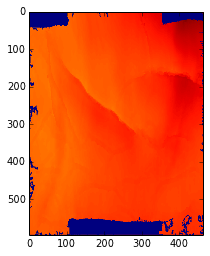Okay, not that easy. There are some problems here:

• It's the wrong shape, because the seismic bins aren't square — we can fix this by setting an aspect ratio
• There's no colourbar — we can add one with plt.colorbar()
• There's not much dynamic range, probably because the nulls are included in the colour range
In :
plt.imshow(horizon, aspect=0.5)
plt.colorbar(shrink=0.75)  # shrink makes the colourbar a bit shorter
plt.show()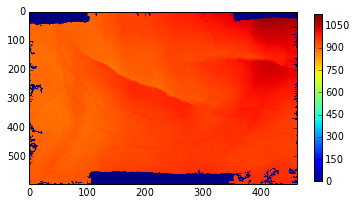This colourmap is no good, rainbows are bad for you. We can switch to a more brain-friendly one called cubehelix (Green, D. A. (2011). A colour scheme for the display of astronomical intensity images. Bulletin of the Astromical Society of India 39, p 289-295. 2011BASI...39..289G and arXiv:1108.5083) and - reverse it by adding _r to the name. We'll set that colourbar as the default:

In :
plt.set_cmap('cubehelix_r')

<matplotlib.figure.Figure at 0x10c6b6250>

As we suspected, the null values are skewing the colourbar. Let's use numpy.percentile to find good values for the min and max. A clever trick lets us exclude datapoints that are zero (or less).

In :
vmin = numpy.percentile(horizon[horizon > 0], 1)
vmax = numpy.percentile(horizon[horizon > 0], 99)
print 'min {0}, max {1}'.format(vmin, vmax)

min 891.804814339, max 1065.95427275


Now we can use these vmin and vmax variables in our plots, so we get nice colours.

In :
plt.imshow(horizon, aspect=0.5, vmin=vmin, vmax=vmax)
plt.colorbar(shrink=0.75)  # shrink makes the colourbar a bit shorter
plt.show()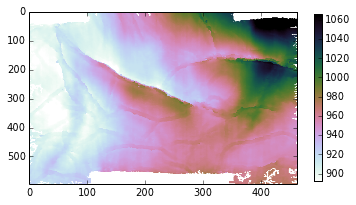These plots aren't interactive, but we can pick an area to replot at a larger scale...

In :
plt.imshow(horizon[200:325,200:300], aspect=0.5, vmin=vmin, vmax=vmax)
plt.colorbar(shrink=0.75)  # shrink makes the colourbar a bit shorter
plt.show()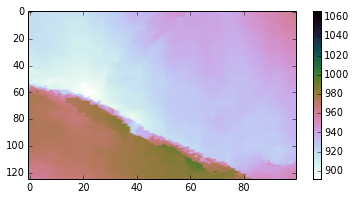Let's show where that is on the main map — I hope ther's a better way to do this, but I'll just draw a bunch of lines...

In :
plt.figure(figsize=(6,3)) # aspect can't cope with the plot overlays
plt.imshow(horizon, vmin=vmin, vmax=vmax)
plt.plot([200,300], [200,200], 'b')
plt.plot([200,300], [325,325], 'b')
plt.plot([200,200], [200,325], 'b')
plt.plot([300,300], [200,325], 'b')
plt.axhline(300, color='k') # another way to draw a line
plt.colorbar()
plt.axis('tight') # makes the plot overlay behave
plt.show()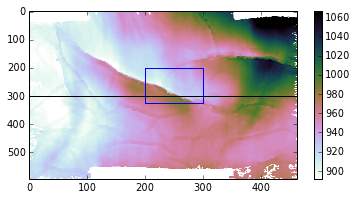This horizon has some edges, which is nice, but it's rather smooth in between the edges. We'd really like to add some noise. the numpy.random library has lots of distributions (Poisson, beta, etc), but we'll just go with a continuous uniform distiubtion, scaled to 100 (otherwise it would be distributed from 0 to 1 ms):

In :
noise = numpy.random.uniform(-15,15, horizon.shape)
noisy_horizon = horizon + noise

plt.figure(figsize=(10,4))
plt.subplot(1,2,1)
plt.imshow(noisy_horizon, aspect=0.5, vmin=vmin, vmax=vmax)
plt.subplot(1,2,2)
plt.imshow(noisy_horizon[200:325,200:300], aspect=0.5, vmin=vmin, vmax=vmax)
plt.show()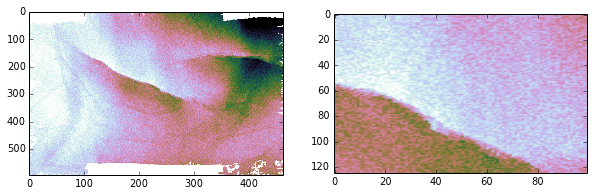Let's look at a 'cross-section' of the horizons together. We'll use slicing to avoid the last few xlines, which have null values and skew the y-axis:

In :
plt.figure(figsize=(15,3))
plt.plot(horizon[300,:450])
plt.plot(noisy_horizon[300,:450], alpha=0.7) # reduce the opacity a bit with alpha
plt.legend(('original', 'noisy'))
plt.gca().invert_yaxis()  # show two-way time increasing downwards
plt.show()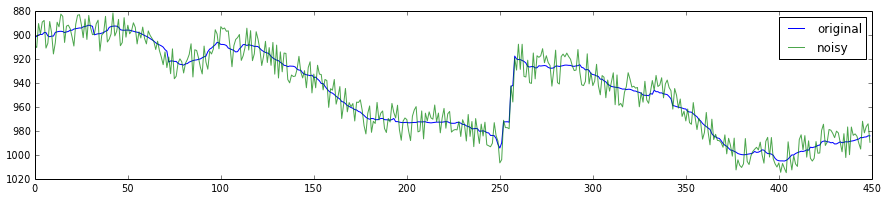# What is convolution?¶

Convolution is an algorithm: a stepwise process with some input and some transformed or generated output. Let's look at a 1D example. We'll convolve a simple pair of numbers, which you can think of as two spikes, with a special function that smoothes spikes out (a filter):

In :
import numpy
my_spikes = numpy.zeros(19)
my_spikes = 1
my_spikes = -2

my_filter = numpy.array([0.05, 0.2, 0.5, 0.2, 0.05])

x = numpy.arange(len(my_spikes)) # for the plot

plt.figure(figsize=(10,3))
plt.subplot(1,2,1)
plt.plot(my_spikes)
plt.subplot(1,2,2)
plt.plot(my_filter)
plt.show()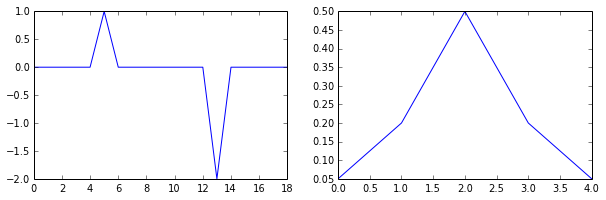Now we can do the convolution. It happens one step at a time. At each step, we multiply the spiky array by the filter, and sum the results. Notice that to evaluate the first element in the array, we'll either need to pad with zeros, or cut off the first two elements. We'll cut them off.

In :
result = numpy.zeros_like(my_spikes[2:-2])  # Copy the 3 to the (n-2)th elements of the array
for i in numpy.arange(len(result)):
for j in numpy.arange(len(my_filter)):
result[i] += my_spikes[(i + 2) +  (j - 2)] * my_filter[j]

print result

plt.plot(my_spikes[2:-2])
plt.plot(result)
plt.show()

[ 0.    0.05  0.2   0.5   0.2   0.05  0.    0.    0.   -0.1  -0.4  -1.   -0.4
-0.1   0.  ]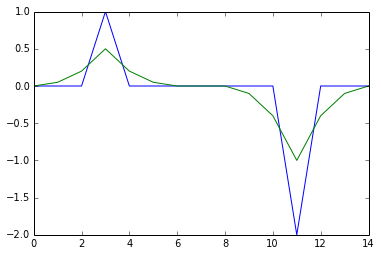The result is essentially just the sum of the kernels, scaled by the spikes.

# Building a kernel¶

Before we can do any work on a horizon, which is a 2D array, we need a 2D kernel. This is the name for the tile, or convolutional operator, we will run over the surface to change it. We will start with the simplest filter: a 3 × 3 mean filter. All we need is an array of 1s, which we divide by 9 for the mean.

In :
kernel = numpy.ones((3,3)) / 9
print kernel

[[ 0.11111111  0.11111111  0.11111111]
[ 0.11111111  0.11111111  0.11111111]
[ 0.11111111  0.11111111  0.11111111]]


A visualization of the kernel is, of course, rather boring:

In :
plt.imshow(kernel, cmap=plt.get_cmap('jet'), interpolation='nearest')
plt.show()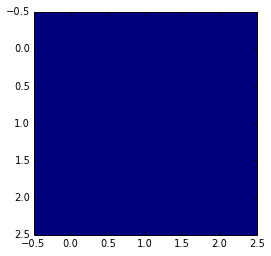# Smooth the data & inspect the output¶

To use the filter, we run it over the surface, one sample at a time. We simply sum the product of the values in the kernel with those in the surface. We'll have to use a nested loop to run across the columns, then down the rows, 'reading' the input with our 'viewfinder' kernel, and 'writing' the output like a book. We'll do some plus and minus 1 and 2 here and there to keep away from the edges. Hmmm, sounds complicated, maybe it's easier to do than to explain:

In :
# make an empty array the same shape as the input
output = numpy.empty_like(horizon)

for x in range(inlines - 2):
for y in range(xlines - 2):
sum_at_this_stop = 0
for i in range(3):
for j in range(3):
horizon_value = noisy_horizon[(x+1)+(i-1),(y+1)+(j-1)]
kernel_value = kernel[i,j]
sum_at_this_stop += kernel_value * horizon_value
output[x+1,y+1] = sum_at_this_stop

plt.imshow(output, aspect=0.5, vmin=vmin, vmax=vmax)
plt.show()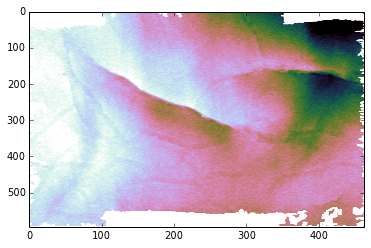To make this tolerate different kernel sizes, we'd want to change the hard-coded offsets (e.g. inlines - 2 and horizon(x+1) — I'm keeping away from the edges) and range(3). The numbers are based on the dimensions of kernel, which we get at using its shape parameter (note the way integers divide here):

In :
print kernel.shape

inline_offset = kernel.shape/2 # this is modulo division, the deault for integers
xline_offset = kernel.shape/2
print inline_offset, xline_offset

(3, 3)
1 1

In :
plt.figure(figsize=(18,4))
plt.plot(horizon[300,:450])
plt.plot(noisy_horizon[300,:450], alpha=0.7)
plt.plot(output[300,:450])
plt.ylim((860,1020))
plt.legend(('original', 'noisy', 'smoothed'))
plt.gca().invert_yaxis()
plt.savefig('Smoothing_result_new.png')
plt.show()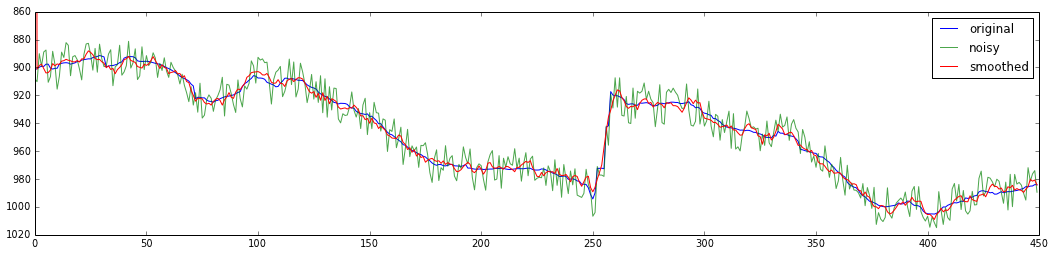It did very well, but did 'erode' the top and bottom of the fault a bit.

# Get faster¶

If this seems a bit, er, complicated, fear not. We don't really have to go through all this. We can just use the extensive SciPy library. NumPy gives us all the n-dimensional array goodness, but SciPy brings the fast Fourier transforms, interpolation, linear algebra, statistics, and signal processing. So compare the crazy nested loop thing above to this:

In :
import scipy.signal
new_output = scipy.signal.convolve2d(noisy_horizon, kernel)

plt.imshow(new_output, aspect=0.5, vmin=vmin, vmax=vmax)
plt.show()